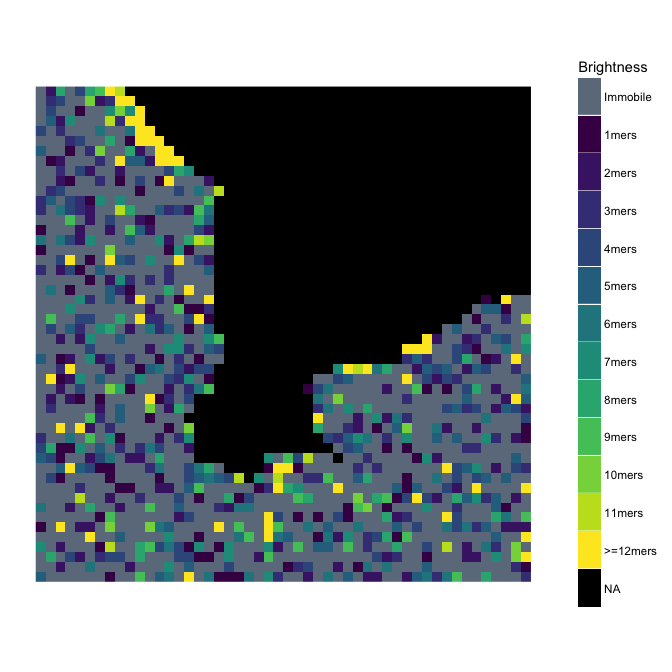# Number and Brightness Image Analysis

#### 2017-07-11

required <- c("nandb", "EBImage", "dplyr", "ggplot2", "matrixStats")
sapply(required, library, character.only = TRUE)
#> Warning: package 'dplyr' was built under R version 3.4.1

Now let’s read in an image:

img <- system.file("extdata", "50.tif", package = "nandb") %>% ReadImageData
dim(img)  # get img's dimensions
#>  50 50 50

So we can see that img is a 3-dimensional array of 70 slices each of which is a $$50 \times 50$$ image. We can view the first slice:

display(normalize(img[, , 1]), method = "raster")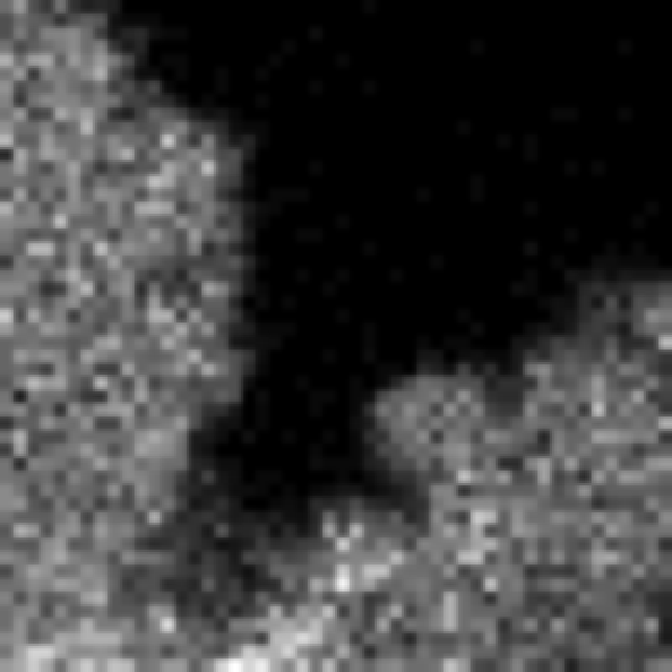### Preprocessing, Brightness Calculation and Post-Filtering

Now we can calculate the brightness image based on this image series, with an exponential filtering detrend with a time constant of (say) 10 frames and using the Huang thresholding method via

img_brightness <- Brightness(img, tau = "auto", mst = "Huang")
MatrixRasterPlot(img_brightness, log.trans = TRUE)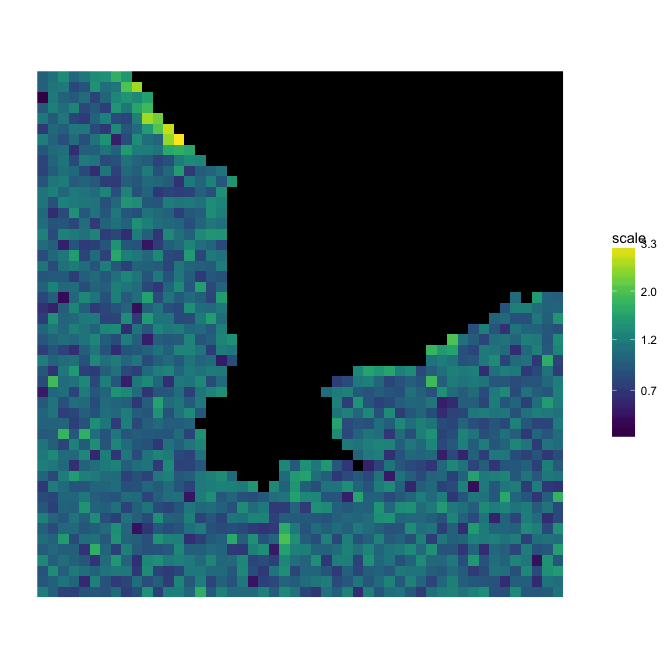and we can see what happens when we median filter.

Brightness(img, tau = "auto", mst = "Huang", filt = "median") %>%
MatrixRasterPlot(scale.name = "brightness", log.trans = TRUE)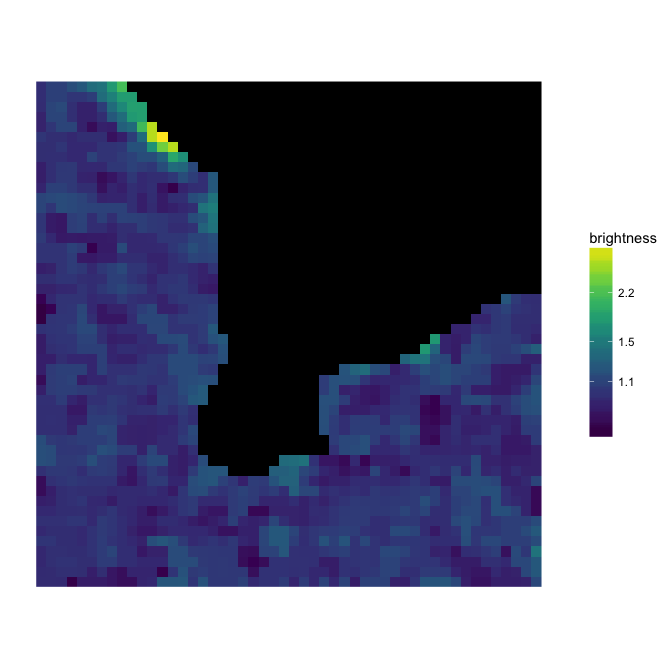### Saving Text Images

We can save the brightness image (let’s do it without median filtering this time). It saves as a text csv file (not as a tiff image, since the brightness values can be any non-negative real number, but tiff images can only store pixel values as integers).

WriteImageTxt(img_brightness, "BrightnessImage")
list.files(pattern = "\\.csv$") # check that the csv file was written to the folder #>  "BrightnessImage.csv" ### Studying the Distribution of Brightnesses We can take a look at the distribution of brightnesses in that image: db <- density(img_brightness, na.rm = TRUE) %>% {data.frame(x = .$x, y = .$y)} ggplot(db, aes(x, y)) + geom_line() + labs(x = "brightness", y = "frequency") + xlim(0.5, 2) #> Warning: Removed 268 rows containing missing values (geom_path).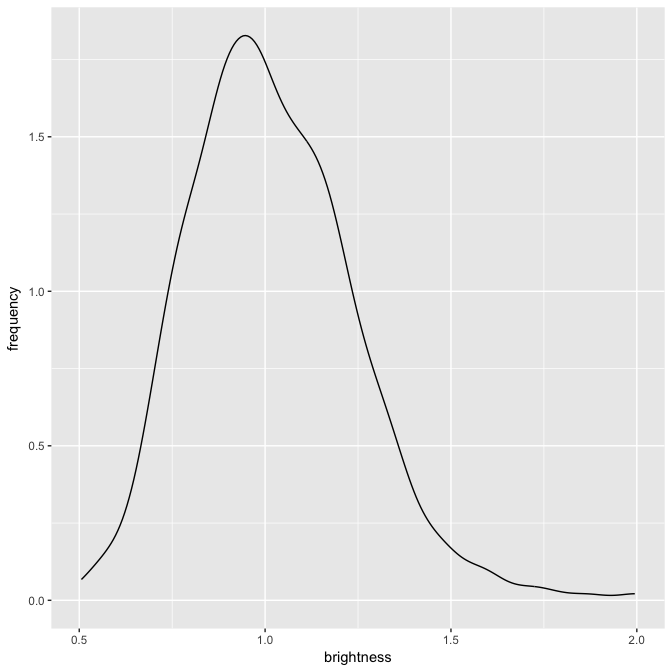and we can compare it to the distribution of brightnesses from immobile particles: immobile_brightnesses <- matrix(rpois(50 * 10^6, 50), nrow = 10^6) %>% {rowVars(.) / rowMeans(.)} di <- density(immobile_brightnesses) %>% {data.frame(x = .$x, y = .\$y)}
rbind(mutate(db, mobility = "mobile"), mutate(di, mobility = "immobile")) %>%      mutate(mobility = factor(mobility)) %>%
ggplot(aes(x, y)) + geom_line(aes(colour = mobility)) +
labs(x = "brightness", y = "frequency") +
xlim(0.5, 2)
#> Warning: Removed 406 rows containing missing values (geom_path).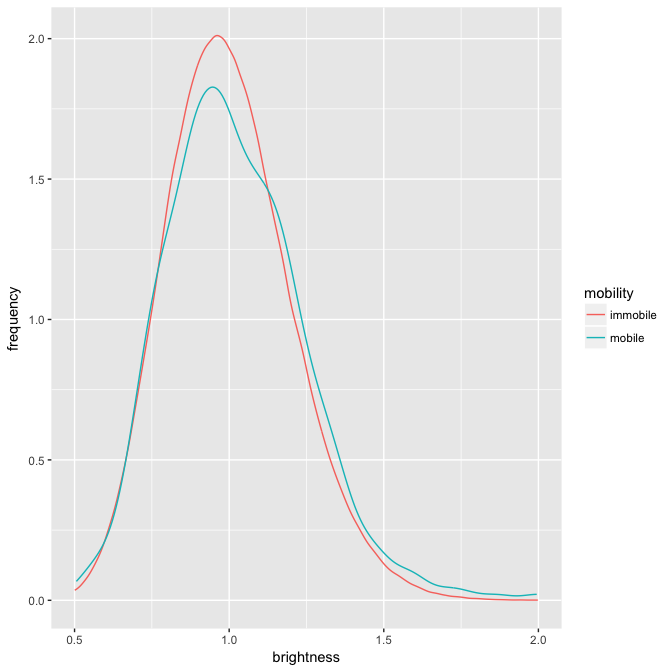### Calculating the Brightness of a Monomer

Say we know that our monomeric brightness is 1.02. Then we can create a plot of all kmers present:

KmerPlot(img_brightness, 1.04)